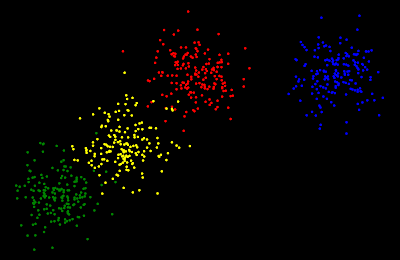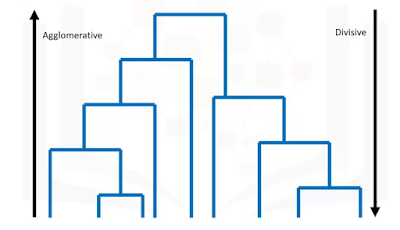## Posts

Showing posts from June, 2020

### Implementing Hierarchical Clustering - In Python Programming languageIntroduction to Hierarchical Clustering Unsupervised learning  is a type of Machine learning in which we use unlabeled data and we try to find a pattern among the data. Clustering algorithms  falls under the category of unsupervised learning. In these algorithms, we try to make different clusters among the data. Hierarchical Clustering  algorithms build a hierarchy of clusters where each node is a cluster consisting of the clusters of its children node. fig. 1 Check out my blog on Hierarchical Clustering - An Unsupervised learning Algorithm  to learn more about it. Implementing it with Python and SciKit-Learn We will use the python programming language for its implementation. In python language, we will be using SciKit-Learn library. So let's start with implementation - We will be using our own dataset which will be generated by us.     1.  Importing necessary Libraries - To create a machine learning model we will use SciKit-Learn (sklearn) library and to create our dataset we will

### Hierarchical Clustering - An Unsupervised Learning AlgorithmIntroduction Unsupervised learning  is a type of Machine learning in which we use unlabeled data and we try to find a pattern among the data. Clustering algorithms falls under the category of unsupervised learning. In these algorithms, we try to make different clusters among the data. Hierarchical Clustering algorithms build a hierarchy of clusters where each node is a cluster consisting of the clusters of its children node. To check it's implementation in Python  CLICK HERE There are various strategies in Hierarchical Clustering such as : Divisive Agglomerative This type of diagram is called  Dendrogram. Divisive - It is a Top-down approach. So we start with all observations in a large cluster and break it down into smaller ones. Agglomerative - It is the opposite of Divisive as it is a Bottom-Up approach. Here, each observation starts in its cluster and pairs of the cluster are merged as they move up the hierarchy.  (Generally, Agglomerative is used more as compared to Divisive

### What is Python ?? || Introduction to Python programmingSource What is Programming?👀💻   In simple language, we can say programming is a technique through which we instruct the computer to perform various tasks .     To instruct computer we need to communicate with a computer and for communication, we need a language as a medium. So programming language serves as a medium of communication between the user and a computer. But, as we know computer only understand binary language and it is practically not possible for us to use binary language with only 0s and 1s.    To solve this problem we need some translator called the compiler. The compiler will compile the code and generate a binary file of it which will be understood by the computer. So this is a complete process of how a computer can be programmed to perform a certain task. Besides compiler there is also an Interpreter. What is Python?🐍   Python is a high level programming language . Designed by Guido Van Rossum. Now a days python is majorly used for DataScience field i.e. Machine L